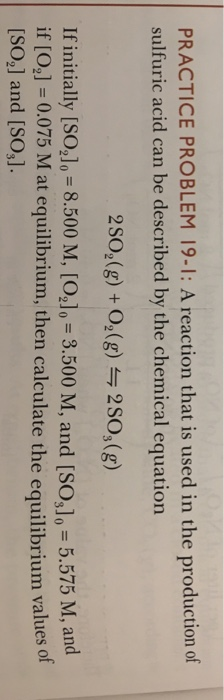# Question & Answer: A reaction that is used in the production of sulphuric acid can be described by the chemical…..A reaction that is used in the production of sulphuric acid can be described by the chemical equation 2SO_2(g) + O_2(g) 2SO_3(g) If initially [SO_2]_0 = 8.500 M, [O_2]_0 = 3.500 M, and [SO_3]_0 = 5.575 M, and if [O_2] = 0.075 M at equilibrium, then calculate the equilibrium values of [SO_2] and [So_3].

Since the concentration of O2 is going to be lower at equilibrium, the equilibrium must shift to the right.
2SO2 + O2 <–> 2SO3

I 8.5M 3.5M 5.575M

Don't use plagiarized sources. Get Your Custom Essay on
Question & Answer: A reaction that is used in the production of sulphuric acid can be described by the chemical…..
GET AN ESSAY WRITTEN FOR YOU FROM AS LOW AS \$13/PAGE

C -2x -x +2x

E 8.5 -2x 3.5 – x 5.575 + 2x

Since the equilibrium of O2 is given, we can solve for x:

3.5-x=0.075
x=3.425

Plug this value into the equilibrium concentrations of So2 and So3:

[so2]= 8.5 – 2(3.425)
= 1.65M

[S03]=5.575 + 2(3.425)
=12.425M